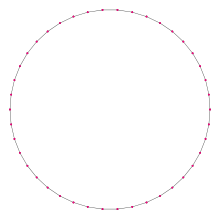Type Regular polygon
Edges and vertices 42
Schläfli symbol {42}, t{21}
Coxeter diagramSymmetry group Dihedral (D42), order 2×42
Internal angle (degrees) ≈171.4286°
Dual polygon Self
Properties Convex, cyclic, equilateral, isogonal, isotoxal

In geometry, a tetracontadigon (or tetracontakaidigon) or 42-gon is a forty-two-sided polygon. (In Greek, the prefix tetraconta- means 40 and di- means 2.) The sum of any tetracontadigon's interior angles is 7200 degrees.

The regular tetracontadigon can be constructed as a truncated icosihenagon, t{21}.

One interior angle in a regular tetracontadigon is 17137°, meaning that one exterior angle would be 847°.

The area of a regular tetracontadigon is (with t = edge length)Since 42 = 2 × 3 × 7, a regular tetracontadigon is not constructible using a compass and straightedge, but is constructible if the use of an angle trisector is allowed.

## Symmetry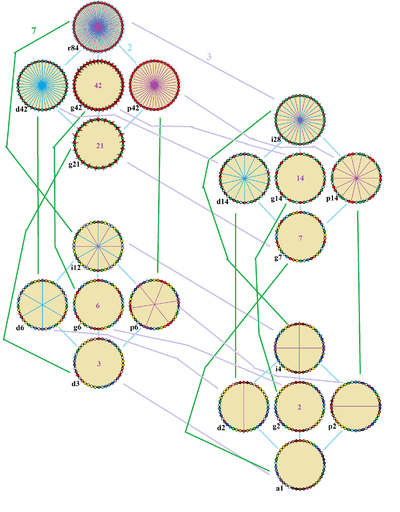The symmetries of a regular tetracontadigon, related as subgroups of index 2, 3, and 7. Lines of reflections are blue through vertices, and purple through edges. Gyrations are given as numbers in the center. Vertices are colored by their symmetry positions.

The regular tetracontadigon has Dih42 dihedral symmetry, order 84, represented by 42 lines of reflection. Dih42 has 7 dihedral subgroups: Dih21, (Dih14, Dih7), (Dih6, Dih3), and (Dih2, Dih1) and 8 more cyclic symmetries: (Z42, Z21), (Z14, Z7), (Z6, Z3), and (Z2, Z1), with Zn representing π/n radian rotational symmetry.

These 16 symmetries generate 20 unique symmetries on the regular tetracontadigon. John Conway labels these lower symmetries with a letter and order of the symmetry follows the letter. He gives r84 for the full reflective symmetry, Dih42, and a1 for no symmetry. He gives d (diagonal) with mirror lines through vertices, p with mirror lines through edges (perpendicular), i with mirror lines through both vertices and edges, and g for rotational symmetry. a1 labels no symmetry.

These lower symmetries allows degrees of freedoms in defining irregular tetracontadigons. Only the g42 subgroup has no degrees of freedom but can seen as directed edges.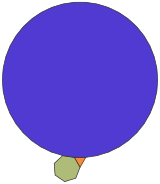An equilateral triangle, a regular heptagon, and a regular tetracontadigon can completely fill a plane vertex. However the entire plane cannot be tiled with regular polygons while including this vertex figure. However it can be used in a tiling with equilateral polygons including rhombi.

 Picture Interior angle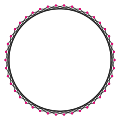{42/5}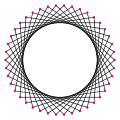{42/11}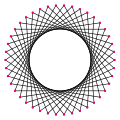{42/13}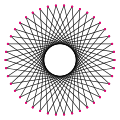{42/17}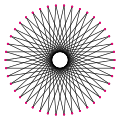{42/19} ≈137.143° ≈85.7143° ≈68.5714° ≈34.2857° ≈17.1429°